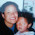## Monday, 3 July 2017

### Extension work.

See if you can solve these word problems. Show how you worked it out!! (use brackets to expand numbers)

This week we have been learning how to solve a stage 7 strategy, I had to explain how I got my answer in brackets.

1. It rained 1.35 inches on Saturday and 1.98 inches on Sunday. How much did it rain on Saturday and Sunday combined? 3.33
(1+1=2)(0.3+0.9=1.2)(0.05+0.08=0.13.)

1. There were 7.6 litres of water in Jacob's bathtub. Then 2.9 litres drained out. How much water is left in the bathtub? 4.7
7.6 - 2.9 = 4.7
(0.7 - 0.2 = 0.5) + (.6 - .9 = 0.3)

1. A carpenter bought a piece of wood that was 6.15 meters long. Then he sawed 2.14 meters off the end. How long is the piece of wood now? 4.01
6.15 - 2.14 = 4.01
(60 - 20 = 40) + (0.1 - 0.1 = 0) + (.5 - .4 = .1)

1. A chef bought 4.2 kilograms of almonds and 7.8 kilograms of pecans. How many kilograms of nuts did the chef buy in all? 12
4.2 + 7.8 = 12
(40 + 70 = 110) + (0.2 + 0.8 = 1)

1. It snowed 8.9 inches on Monday and 3.9 inches on Tuesday. How much did it snow on Monday and Tuesday combined? 12.8
8.9 + 3.9 = 12.8
(80 + 30 = 110) + (0.9 + 0.9 = 1.8)

1. It rained 0.97 cm on Monday. On Tuesday, it rained 0.4 cm less than on Monday. How much did it rain on Tuesday? READ CAREFULLY-THIS ONE WILL TRY AND TRICK YOU!  1.05

0.97 - 0.4 = 1.05
(0 - 0 = 0) + (0.9 - 0.4 = 0.5) + .7

1. Omar spent Saturday picking berries at a berry farm. In the morning, he picked 3.61 kg of berries. In the afternoon, he picked 2.4 kgs. How many pounds of berries did Omar pick in all? 6.01
3.61 + 2.4 = 6.01
(30 + 20 = 50) + (0.6 + 0.4 = 1) + .1

1. A bee colony produced 0.91 pounds of honey, but bears ate 0.9 pounds of it. How much honey remains? 0.01
0.91 - 0.9 = 0.01
(0 - 0 = 0) + (0.9 - 0.9 = 0) + .9

1. Oscar bought 5.87 pounds of melon and 3.18 pounds of cherries. How much fruit did he buy in all? 9.05
5.87 + 3.18 = 9.05
(50 + 30 = 80) + (0.8 + 0.1 = 0.9) + (.7 + .8 = 1.5)

1. Vince mixed 0.8 grams of salt into a pot of soup he was cooking. Before he served the soup, Vince added 0.25 grams of salt. How much salt did Vince put into the soup in all? 1.05
0.8 + 0.25 = 1.05

(0 + 0 = 0) + (0.8 + 0.2 = 1) + .5

#### 1 comment:

1.Wow Honey - Math sure has changed from when i was in school. Great job on this :)